Filed under:

# Take the Wonderlic Test

When Terrelle Pryor's Wonderlic scores were circulating in the news, it got me thinking. You always hear about the Wonderlic test, but you never see one. At least I hadn't. So I went in search of a test. I couldn't find the official test, however, I did find a sample test.

The official Wonderlic is a 50 question 12-minute test. What we have here, courtesy of WalterFootball.com, is a 20 question version. So, give yourself five minutes and then mulitiply your correct answer(s—I hope) by 2.5.

Note: I scored a 96. If you're wondering how I got a 96 out of a possible 50, don't bother. You have to score a 96 to be smart enough to figure it out.

According to Wiki, average intelligence equates to 20. The average score for a chemist is 31, for a football player its 21, a warehouse worker's average is 14.

The answer key is at the bottom. Set your timer and jump over to take the test.

• Look at the row of numbers below. What number should come next?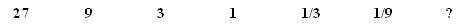• Assume the first 2 statements are true. Is the final one:The boy plays for the Tigers. All the players on the Tigers wear white sneakers. The boy wears white sneakers.

• Paper sells for 21 cents per pad. What will 5 pads cost?

• How many of the six pairs of items listed below are exact duplicates?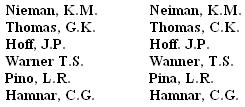• PRESENT, RESENT - Do these words• A train travels 20 feet in 1/5 seconds At this exact speed, how many feet will it travel in three seconds?

• When rope is selling at \$.10 a foot, how many feet can you buy for sixty cents?

• The eighth month of the year is:• Which number in the following group of numbers represents the smallest amount?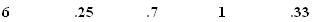• In printing an article of 48,000 words, a printer decides to use two sizes of type. Using the larger type, a printed page contains 1,800 words. Using smaller type, a page contains 2,400 words. The article is allotted 21 full pages in a magazine. How many pages must be in smaller type?

• Three individuals form a partnernship and agree to divide all profits equally. X invests \$9,000, Y invests \$7,000 and Z invests \$4,000. If the profits are \$4,800, how much less does X receive than if the profits were divided in proportion to the amount invested?

• Assume the first 2 statements are true. Is the final one:Ben greeted Beth. Beth greeted Bob. Ben did not greet Bob.

• A boy is 16 years old and his sister is twice as old. When the boy is 22 years old, what will be the age of his sister?

• One of the numbered figures in the following drawing is most different from the others. What number is that figure?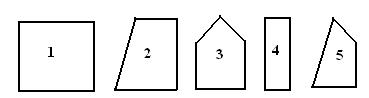• The hours of daylight and darkness in SEPTEMBER are nearest equal to the hours of daylight and darkness in:• What number is next in the following sequence: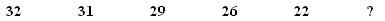• Which of the following numbers is least like the others?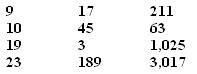• Which letter is missing from the group below?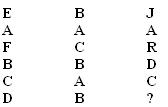• In a typical city in the Southern Hemisphere, which of the following months sees the most snowfall?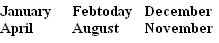• FLAMMABLE, INFLAMMABLE - Do these words• 1/27. Every number is divided by 3 in the sequence.

• 1 - True. All Tigers wear white sneakers and the boy is a Tiger.

• \$1.05. 21 cents x 5 = 105 cents = \$1.05.

• One. Look closely - the I and E are switched in the first; the G becomes a C in the second; the comma after Hoff is replaced with a period in the third; the R in Warner becomes an N in the fourth; and Pino is Pina in the fifth. The sixth is identical.

• 3 - Mean neither the same nor opposite. Look the words up in the dictionary. Nothing alike.

• 300 feet. 20 feet in 1/5 seconds becomes 100 feet in one second (20 x 5 = 100.) So, 100 feet in one second is 300 in three (100 x 3 = 300.)

• 6 feet. 10 cents a foot, 20 cents for 2 feet, 30 cents for 3 feet, etc.

• August. The eighth month of the year is August.

• .25. The closest number to zero in the group is .25.

• 17. We want to maximize the smaller-type pages because using the larger type would use too many pages. So, try using all small type. That would be 2,400 words x 21 pages = 50,400 words, which would leave too much white space. Because the LCD between 1,800 and 2,400 is 7,200, take out 4 pages for use of the larger type (1,800 x 4 = 7,200). That leaves 17 smaller-type pages; 17 x 2,400 = 40,800. Add 7,200 to that (from the larger type) and you get 48,000 words. Hardest question on this test.

Note - I recently received an e-mail from Kristin P from Oregon. She's a print designer, and she had an alternate answer:

Anyway, when I saw the answer to #10, I knew I had to disagree with the "mathematical" solution of 17 pages. Any good print designer would know the immediately PRACTICAL answer to this was 20 pages because 2400 x 20 = 48000 then you intersperse one page worth of subheads in the larger font size, change the tracking and kearning as necessary and make it all fit!!!

Hey, I can't argue with a print designer. 20 pages it is.

• \$560. If the profits are split equally, each guy gets \$4,800/3 = \$1,600. If the profits are split proportionally, X would receive 45 percent (\$9,000/(\$9,000 + \$7,000 + \$4,000). So, 45 percent of \$4,800 is .45 x \$4,800 = \$2,160. Just subtract \$1,600 from \$2,160 and you get \$560.

• 3 - Not certain. Just because Ben met Beth, and Beth met Bob, we don't know if Ben even knows Bob.

• 38. If the sister is twice as old, she's 32. The boy will be 22 six years from now, so 32 + 6 = 38.

• 3. All of the figures have four sides, with the exception of No. 3.

• March. March and September have equal amounts of sunlight and darkness on the 21st of each month.

• 17. 32-1 = 31. 31-2 = 29. 29-3 = 26. 26-4 = 22. So, the next thing you subtract is 5. 22-5 = 17.

• 10. All of the other numbers are odd.

• H. Replace A with 1, B with 2, C with 3, etc. Multiply the first column by the second column to get the third. D = 4 and B = 2, so 4x2= 8. The eighth letter of the alphabet is H.

• August. The Southern Hemisphere is opposite of the Northern, so our summer is their winter. August is the only month listed remotely close to our summer.

• 1 - Have similar meanings. Flammable and inflammable mean the exact same thing.